# Birthdays

In the classroom, students always give candy to their classmates on their birthdays. The birthday person always gives each one candy, and he does not give himself. A total of 650 candies were distributed in the class per year. How many students are in the class? (Note: All students in the class had a birthday on the day of the class. )

n =  26

### Step-by-step explanation:Did you find an error or inaccuracy? Feel free to write us. Thank you!Tips to related online calculators
Looking for help with calculating roots of a quadratic equation?
Do you have a linear equation or system of equations and looking for its solution? Or do you have a quadratic equation?

## Related math problems and questions:

• School year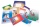At the beginning of the school year, 396 notebooks and 252 textbooks are ready to be distributed in the classroom. All pupils receive the same number of notebooks and the same amount of textbooks. How many pupils are there in the class if you know that th
• CandiesIf Alena give Lenka 3 candy will still have 1 more candy. If Lenka give Alena 1 candy Alena will hame twice more than Lenka. How many candies have each of them?
• Average height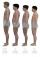In a class are 34 students. The average height of the students is 165 cm. What will be the average height of students in the classroom when two pupils, tall 176 cm and 170 cm, moved from this school/class? The average height in meters of all the students
• Candy and boxesWe have some candy and empty boxes. When we put candies in boxes of ten, there will be 2 candies and 8 empty boxes left. When of eight, there will be 6 candies and 3 boxes left. How many candy and empty boxes left when we put candies in boxes of nine?
• GrandmotherGrandmother wants to give the candies to grandchildren that when she gives 5 candy everyone 3 missing and when she gives 4 candies 3 is surplus. How many grandchildren has grandmother and how many candies has?
• Marks at schoolThere are 30 students in the class. Five students in the class had a mark three triple at the end of the math certificate, and the other students had a mark of one or two. The average mark in all students' mathematics in the class at the end of the year w
• Jane classWhen asked how many students are in class, Jane said, if we increase the number of students in our class by hundred % and then add half the number of students, we get 100. How many students are in Jane's class?
• CandyPeter had a sachet of candy. He wanted to share with his friends. If he gave them 30 candies, he would have 62 candies. If he gave them 40 candies, he would miss 8 candies. How many friends did Peter have?
• Exp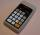If sqrt(202-122) = sqrt[n](256), then n is:
• Car factory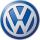The carmaker now produces 2 cars a day more than last year, so the production of 70312 cars will save just one full working day. How many working days needed to manufacture 70312 cars last year?
• CandiesThe price for 1 kg of more expensive candies is 125 CZK. The price for 1 kg of cheaper candy is 100 CZK. We mix two different mixtures of candy. A) The first mixture contains 2 kg more expensive and 0.5 kg cheaper candy. Calculate the price for 1 kg of th
• SweetsWe want to prepare 5 kg of sweets for 150 CZK. We will mix cheaper candy: 1 kg for 120 CZK and more expensive candy: 1 kg per 240 CZK. How much of these two types of candy is necessary to prepare this mixture?
• School booksAt the beginning of the school year, the teacher distributed 480 workbooks and 220 textbooks. How many pupils could have the most in the classroom?
• Collecting day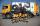On collecting day at school, pupils of three classes took a total of 1063 kg of paper. Class B collected half more than A-Class and class C 55 kg more than B. How many kg of paper collected in each class?
• BonbonsCreate a mixture of 50 kg of candy on price 700Kč. Candies has prices: 820Kč, 660Kč and 580Kč. Use cross rule.
• Automaker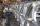The automaker now produces daily 4 new cars more than last year so the production of 360 cars will save just one full working day. How many working days to produce 360 vehicles needed last year?Solve quadratic equation: 2x2+28x-550=0